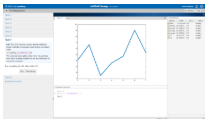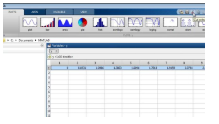# MATLAB 快速入门

MATLAB 可帮助您不仅仅将自己的创意停留在桌面。您可以对大型数据集运行分析，并扩展到集群和云。MATLAB 代码可以与其他语言集成，使您能够在 Web、企业和生产系统中部署算法和应用程序。

## 教程

• 桌面基础知识

在命令行上输入语句并查看结果。

• 矩阵和数组

MATLAB 主要处理数组和矩阵，既可以整个处理，也可以部分处理。矩阵是指通常用来进行线性代数运算的二维数组。

• 数组索引

MATLAB 中的变量通常是可包含很多数字的数组。如果要访问数组的选定元素，请使用索引。

• 工作区变量

工作区包含在 MATLAB 中创建或从数据文件或其他程序导入其中的变量。

• 文本和字符

为文本创建字符串数组，或为数据创建字符数组。

• 调用函数

MATLAB 提供了大量执行计算任务的函数。要调用函数，请将其输入参数括在圆括号中。

• 二维图和三维图

图形函数包括二维和三维绘图函数，用于以可视化形式呈现数据和通信的结果。

• 编程和脚本

最简单的一种 MATLAB 程序称为脚本。脚本包含一系列命令和函数调用。

• 帮助和文档

所有函数都有辅助文档，这些文档包含一些示例，并介绍函数输入、输出和调用语法。

• 矩阵和幻方矩阵

输入矩阵，执行矩阵运算并访问矩阵元素。

• 表达式

使用变量、数字、运算符、函数和表达式。

• 输入命令

更改输出格式，隐藏输出，输入长行，以及在命令行中进行编辑。

• 索引

访问矩阵元素，通过串联创建矩阵，以及删除矩阵行和列。

• 数组类型

使用多维数组，元胞数组，字符与文本数据以及结构体。

• 线性代数

本主题介绍如何在 MATLAB 中创建矩阵和执行基本矩阵计算。

• 非线性函数的运算

可以创建任何 MATLAB 函数的句柄，并将该句柄用作引用该函数的一种方式。函数句柄通常在参数列表中传递给其他函数，然后，其他函数可以使用该句柄执行或计算相应函数。

• 多变量数据

MATLAB 对多变量统计数据使用列向分析。数据集中的每一列都代表一个变量，每一行都代表一个观测值。第 `(i,j)` 个元素是第 `j` 个变量的第 `i` 个观测值。

• 基本绘图函数

使用绘图函数创建和修改绘图。

• 创建网格图和曲面图

可视化包含两个变量的函数。

• 显示图像

使用图像。

• 打印图形

打印和导出图窗。

• 处理图形对象

可视化包含两个变量的函数。

• 控制流

使用流控制结构，包括 `if``switch``case``for``while``continue``break`

• 脚本和函数

编写脚本和函数，使用全局变量，向函数传递字符参数，使用 `eval` 计算文本表达式，向量化代码，预分配数组，使用句柄引用函数，以及使用处理函数的函数。

## 交互式学习MATLAB 入门之旅

## 视频MATLAB 快速入门